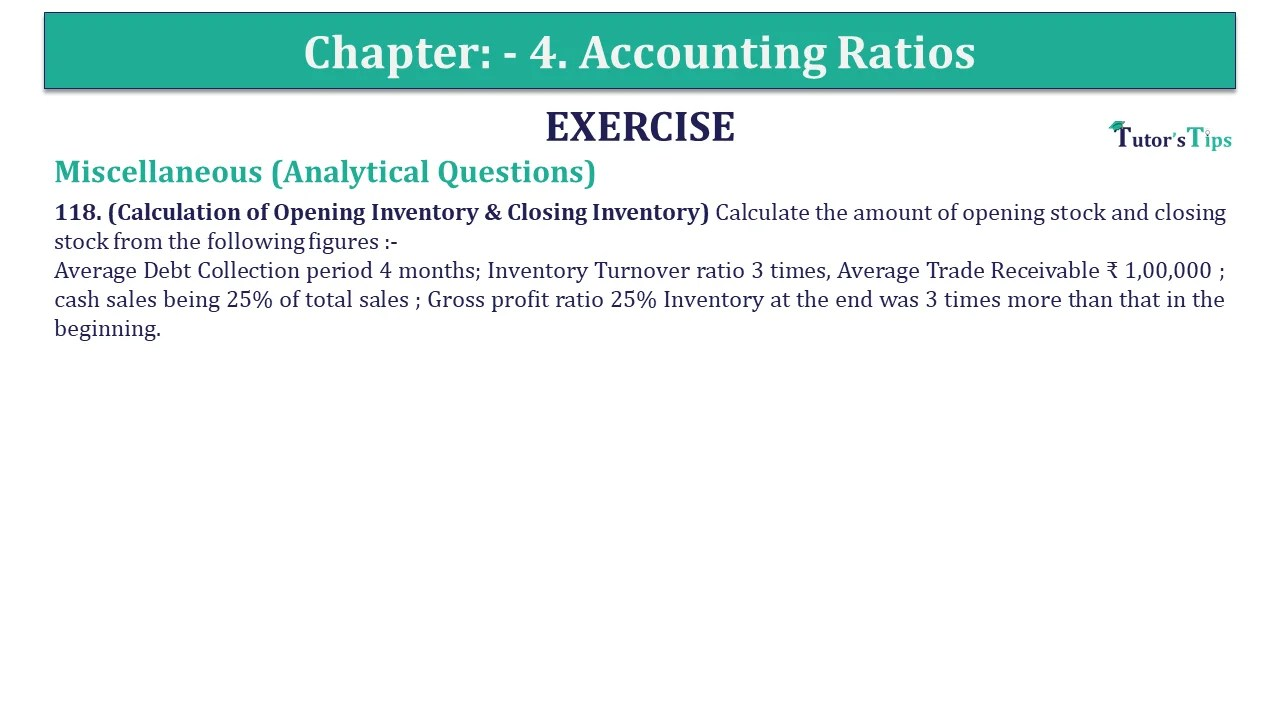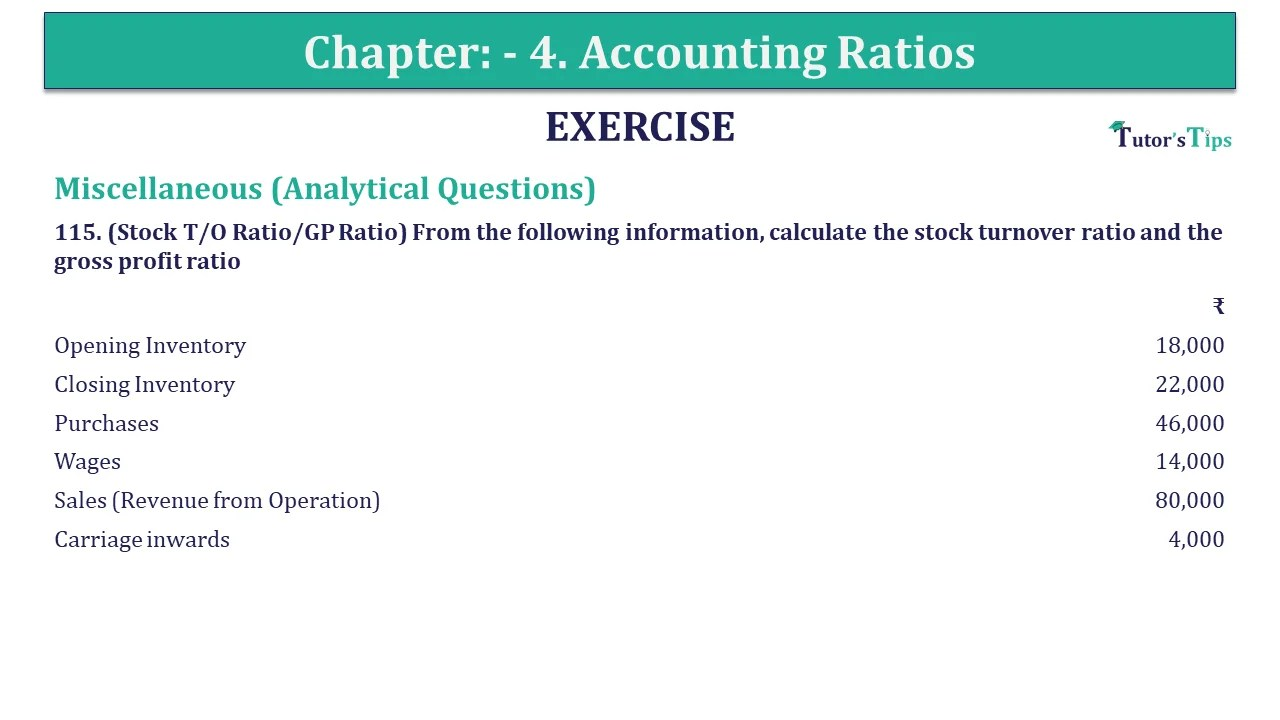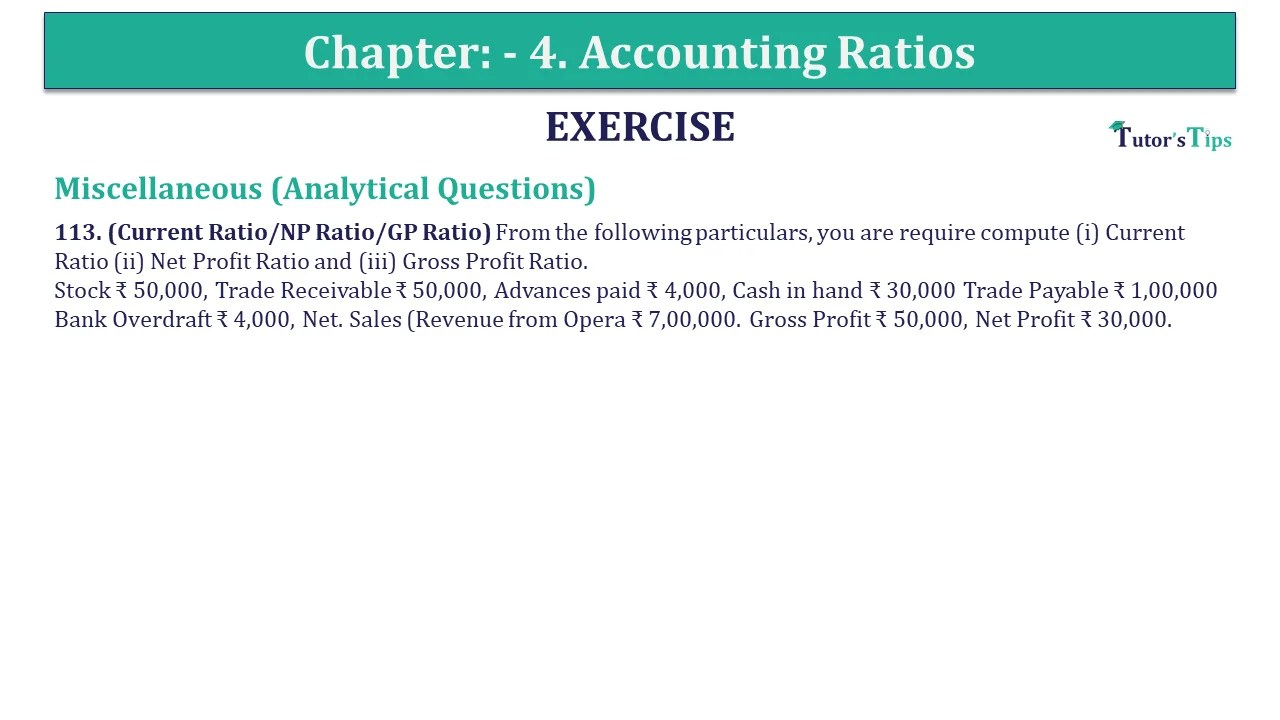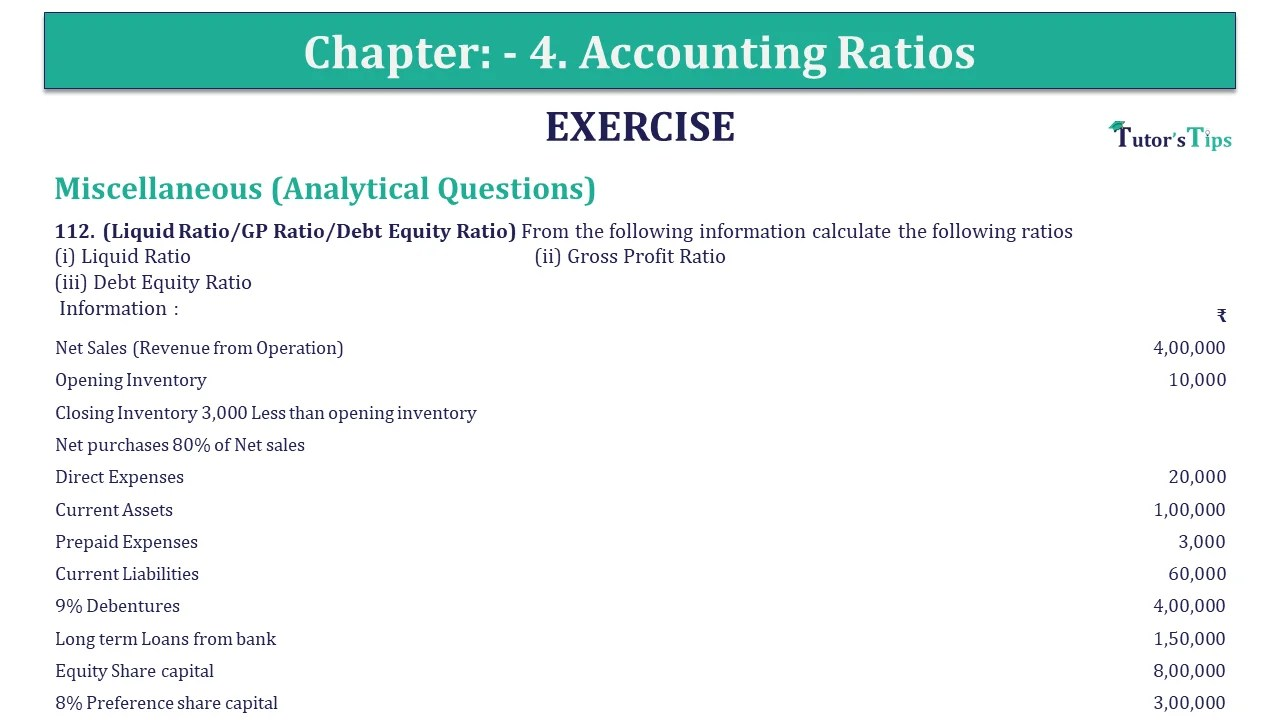## Question 121 Chapter 4 of +2-B – USHA Publication 12 ClassQuestion 121 Chapter 4 of +2-B Miscellaneous (Analytical Questions) 121. (GP Ratio/Inventory T/0 Ratio/Operating Ratio) From the details given below, calculate the following ratios: (a) Gross Profit Ratio (b) Inventory Turnover Ratio (c) Operating Ratio   ₹ Sales (Revenue from Read More …

## Question 120 Chapter 4 of +2-B – USHA Publication 12 ClassQuestion 120 Chapter 4 of +2-B Miscellaneous (Analytical Questions) 120. (Return on Capital Employed) From the following Balance sheet of ABC Ltd. as on 31st March 2018. BALANCE SHEET  Particulars  ₹ I. Equity and Liabilities   Shareholders’ Funds   Share Read More …

## Question 119 Chapter 4 of +2-B – USHA Publication 12 ClassQuestion 119 Chapter 4 of +2-B Miscellaneous (Analytical Questions) 119. (Operating Ratio/Liquid Ratio/Proprietary Ratio) From the following information calculate the (a) Operating ratio (b) Liquid Ratio (c) Proprietary Ratio Information : Cash Sales (Revenue from Operation) ₹ 4,00,000, Credit Sales Read More …

## Question 118 Chapter 4 of +2-B – USHA Publication 12 ClassQuestion 118 Chapter 4 of +2-B Miscellaneous (Analytical Questions) 118. (Calculation of Opening Inventory & Closing Inventory) Calculate the amount of opening stock and closing stock from the following figures:- Average Debt Collection period 4 months; Inventory Turnover ratio 3 Read More …

## Question 117 Chapter 4 of +2-B – USHA Publication 12 ClassQuestion 117 Chapter 4 of +2-B Miscellaneous (Analytical Questions) 117. (Quick Ratio/Inventory T/O Ratio/Return on Shareholders’ Investment) The following are the Summarised Statement of Profit and Loss of Hindustan Products for the year ended 31.3.2018 and the Balance sheet of Read More …

## Question 116 Chapter 4 of +2-B – USHA Publication 12 ClassQuestion 116 Chapter 4 of +2-B Miscellaneous (Analytical Questions) 116. (GP Ratio/Current Ratio/Liquid Ratio) Following is the Profit and Loss Account and Balance Sheet of New Company Ltd. for the year ending 31st March, 2018. STATEMENT OF PROFIT AND LOSS  Read More …

## Question 115 Chapter 4 of +2-B – USHA Publication 12 ClassQuestion 115 Chapter 4 of +2-B Miscellaneous (Analytical Questions) 115. (Stock T/O Ratio/GP Ratio) From the following information, calculate the stock turnover ratio and the gross profit ratio   ₹ Opening Inventory 18,000 Closing Inventory 22,000 Purchases  46,000 Wages  14,000 Read More …

## Question 114 Chapter 4 of +2-B – USHA Publication 12 ClassQuestion 114 Chapter 4 of +2-B Miscellaneous (Analytical Questions) 114. (GP Ratio/Inventory T/O Ratio/Trade Receivable T/O Ratio/Net Profit Ratio) The following information is given about a company :   ₹ Sales (Revenue from Operation) 4,00,000 Gross profit 10,000 Cost of Read More …

## Question 113 Chapter 4 of +2-B – USHA Publication 12 ClassQuestion 113 Chapter 4 of +2-B Miscellaneous (Analytical Questions) 113. (Current Ratio/NP Ratio/GP Ratio) From the following particulars, you are required to compute (i) Current Ratio (ii) Net Profit Ratio and (iii) Gross Profit Ratio.Stock ₹ 50,000, Trade Receivable ₹ Read More …

## Question 112 Chapter 4 of +2-B – USHA Publication 12 ClassQuestion 112 Chapter 4 of +2-B Miscellaneous (Analytical Questions) 112. (Liquid Ratio/GP Ratio/Debt Equity Ratio) From the following information calculate the following ratios (i) Liquid Ratio (ii) Gross Profit Ratio (iii) Debt Equity Ratio Information :   ₹ Net Sales Read More …